# Why histograms¶

As we all know, Histograms are an extremely common way to make sense of discrete data. Whether we mean to or not, when we're using histograms, we're usually doing some form of density estimation. That is, although we only have a few discrete data points, we'd really pretend that we have some sort of continuous distribution, and we'd really like to know what that distribution is. For instance, I was recently grading an exam and trying to figure out what the underlying distribution of grades looked like, whether I should curve the exam, and, if so, how I should curve it.

I'll poke at this in an IPython Notebook; if you're doing this in a different environments, you may wish to uncomment out the commented lines so that your namespace is properly polluted.

In :
from __future__ import division
%pylab inline


Populating the interactive namespace from numpy and matplotlib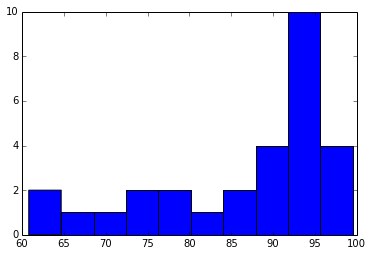# Why not histograms?¶

We can play around with the number of bins, but it's not totally clear what's going on with the left half of the grades.

In :
junk = hist(grades,5)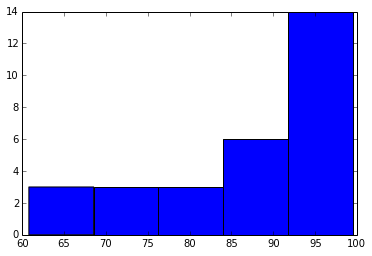In :
junk = hist(grades,15)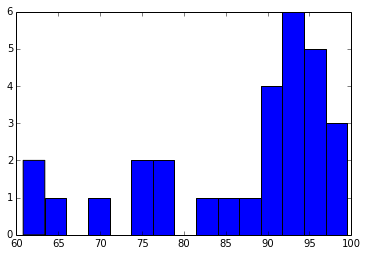So, maybe the histogram isn't the perfect tool for the job at hand. In fact, there are quite a few well-known problems with histograms. Shodor has a really nice histogram activity that lets you play around with data interactively. Rather than using Java or JavaScript directly, Jake Vanderplas has a great package called JSAnimation that lets us animate things directly in IPython Notebooks. I'll cheat a bit: since all I really need for this is a single slider, I can use JSAnimation to let us interact with data very similarly to the Shodor pages.

In :
from JSAnimation.IPython_display import display_animation, anim_to_html


Before we start, I'll load in a few data sets. If you're interested, you can rerun this notebook with a different data set to see how it affects things. data_shodor is the "My Data" set from their histogram activity page, data_sat is the average SAT Math data from the same page, data_tarn is from Tarn Duong's fantastic KDE explanation (we'll get there), and simple_data is just a very simple data set.

In :
data_tarn = array((2.1,2.2,2.3,2.25,2.4,2.61,2.62,3.3,3.4,3.41,3.6,3.8))
data_shodor = array((49,49,45,45,41,38,38,38,40,37,37,34,35,36,35,38,38,32,32,32,37,31,32,31,32,30,30,32,30,30,29,28,29,29,29,30,28,27,29,30,28,27,28,27,27,29,29,29,26,27,25,25,25,25,25,25,25,26,26,27))
data_sat = array((490,499,459,575,575,513,382,525,510,542,368,564,509,530,485,521,495,526,474,500,441,750,495,476,456,440,547,479,501,476,457,444,444,467,482,449,464,501,670,740,590,700,590,450,452,468,472,447,520,506,570,474,532,472,585,466,549,736,654,585,574,621,542,616,547,554,514,592,531,550,507,441,551,450,548,589,549,485,480,545,451,448,487,480,540,470,529,445,460,457,560,495,480,430,644,489,506,660,444,551,583,457,440,470,486,413,470,408,440,596,442,544,528,559,505,450,477,557,446,553,370,533,496,513,403,496,543,533,471,404,439,459,393,470,650,512,445,446,474,449,529,538,450,570,499,375,515,445,571,442,492,456,428,536,515,450,537,490,446,410,526,560,560,540,502,642,590,480,557,468,524,445,479))
simple_data = array((0,5,10))


Two of the main problems with histograms are (1) you need to define a bin size (2) you need to decide where the left edge of the bin is.

## Histogram bin size¶

Let's look at the effects of bin size on histograms.

Caveat: the code below is certainly not optimized. Ditto for all of the code in this notebook. I wrote it quickly and at the same time I learned what FuncAnimation does. In order to make this read more easily, I've included most of the code at the end. If you're running this interactively, run the cell at the end now!

Let's start with getHistBinNumAni. What does that do? Given a data set, it'll give us an interactive plot. By dragging the slider around, we can make a histogram with anywhere from 1 bin to some max (default: 20) number of bins. No matter how many bins we have, the actual data is shown in blue dots near the bottom. Here's what it looks like for the grades:

In :
ani = getHistBinNumAni(data)
display_animation(ani, default_mode='once')

Out:

Once Loop Reflect

So, obviously chosing the number of bins makes a huge difference in how we'd interpret the data.

## Where do the histogram bins start?¶

One of the other big problems with histograms, especially for relatively small data sets, is that you have to choose where the left edge of the first bin goes. Do you center the bin around the first group of points? Do you make the left edge match up with the left-most data point? Let's make some plots to see how that can affect things, because it's a bit easier to understand what I'm going on about that way. We'll make a similar animation with getHistBinOffsetAni. As with the previous animation, drag the slider around. This time, we have the same number of bins, but the slider drags around the data relative to the bins (or vice versa, depending on how you think of it).

In :
ani = getHistBinOffsetAni(data)
display_animation(ani, default_mode='once')

Out:

Once Loop Reflect

# KDE (Kernel Density Estimation) to the rescue!¶

Kernel density estimation is my favorite alternative to histograms. Tarn Duong has fantastic KDE explanation, which is well worth reading. The basic idea is that, if you're looking at our simple dataset (simple_data = array((0,5,10)), you might choose to represent each point as a rectangle:

In :
bar(simple_data,ones_like(simple_data)*0.5,width=0.5,facecolor='grey',alpha=0.5)
junk = ylim(0, 2.0)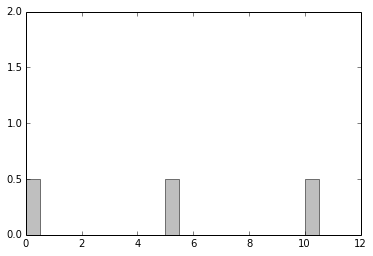not so interesting so far, but what do we do when the rectangles get wide enough that they start to overlap? Instead of just letting them run over each other like

In :
bar(simple_data,ones_like(simple_data)*0.5,width=6,facecolor='grey',alpha=0.5)
junk = ylim(0, 2.0)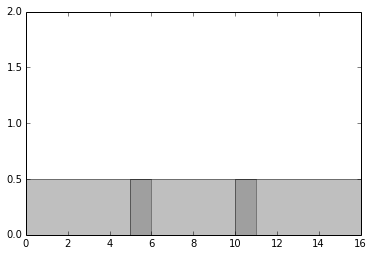and instead of coloring the overlap regions darker grey, we add the rectangles together. So, since each of the rectangles has height 0.5 in the above example, the dark grey regions should really have height 1.0. This idea is called "kernel density estimation" (KDE), and the rectangle that we're using is called the "kernel". If we wanted to draw a different shape at each point, we'd do so by specifying a different kernel (perhaps a bell curve, or a triangle).

## KDE, rectangular kernel¶

Now let's try KDE with a rectangular kernel. This time, using getKdeRectAni, you get a slider controls the width of the kernel.

In :
ani = getKdeRectAni(simple_data)
display_animation(ani, default_mode='once')

Out:

Once Loop Reflect